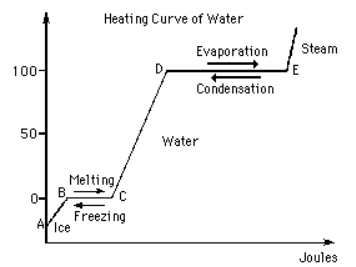# Problem: The graph below shows the heating curve of water. One can plot heating curves by measuring the increase in temperature of a given amount of ice, water or steam as a function of heat that is added at a constant rate. The first slope (from A to B) refers to the change in temperature of ice as heat is added. At the melting point of ice (B) the temperature remains constant until all of the ice is melted. The graph between C and D represents the increase in the temperature of water, until the boiling point is reached (D). At the boiling point the temperature of the water remains constant until all of the water is converted into steam (from D to E). The last portion of the curve (from E) represents the heating of steam.Determine if the following statements are True or False.Select all that are True.1. Evaporation is an endothermic process because the system is absorbing energy.2. Cooling water is an exothermic process which decreases the internal energy of the system.3. Hydrogen bonds between water molecules are broken when evaporation occurs. (From D to E)4. As water is heated, the system is absorbing energy from the surroundings.5. At the boiling point of water (1 atm and 373 K) water and steam are in equilibrium with each other.6. Heating ice (going from A to B), increases the vibrations of the atoms.7. Heating water (from C to D), decreases the kinetic energy of water.8. The freezing of water is an endothermic process because the system absorbs energy.

###### FREE Expert Solution
81% (473 ratings)
###### FREE Expert Solution

The diagram is a heating curve where a certain substance, water in this case, is being observed when temperature is increased. Changes in phase and corresponding energy will be analyzed for diagrams like this.

81% (473 ratings)###### Problem Details

The graph below shows the heating curve of water. One can plot heating curves by measuring the increase in temperature of a given amount of ice, water or steam as a function of heat that is added at a constant rate. The first slope (from A to B) refers to the change in temperature of ice as heat is added. At the melting point of ice (B) the temperature remains constant until all of the ice is melted. The graph between C and D represents the increase in the temperature of water, until the boiling point is reached (D). At the boiling point the temperature of the water remains constant until all of the water is converted into steam (from D to E). The last portion of the curve (from E) represents the heating of steam.

Determine if the following statements are True or False.

Select all that are True.
1. Evaporation is an endothermic process because the system is absorbing energy.
2. Cooling water is an exothermic process which decreases the internal energy of the system.
3. Hydrogen bonds between water molecules are broken when evaporation occurs. (From D to E)
4. As water is heated, the system is absorbing energy from the surroundings.
5. At the boiling point of water (1 atm and 373 K) water and steam are in equilibrium with each other.
6. Heating ice (going from A to B), increases the vibrations of the atoms.
7. Heating water (from C to D), decreases the kinetic energy of water.
8. The freezing of water is an endothermic process because the system absorbs energy.What scientific concept do you need to know in order to solve this problem?

Our tutors have indicated that to solve this problem you will need to apply the Heating and Cooling Curves concept. You can view video lessons to learn Heating and Cooling Curves. Or if you need more Heating and Cooling Curves practice, you can also practice Heating and Cooling Curves practice problems.

What is the difficulty of this problem?

Our tutors rated the difficulty ofThe graph below shows the heating curve of water. One can pl...as medium difficulty.

How long does this problem take to solve?

Our expert Chemistry tutor, Dasha took 8 minutes and 36 seconds to solve this problem. You can follow their steps in the video explanation above.

What professor is this problem relevant for?

Based on our data, we think this problem is relevant for Professor Habel Rodriguez's class at UNM.